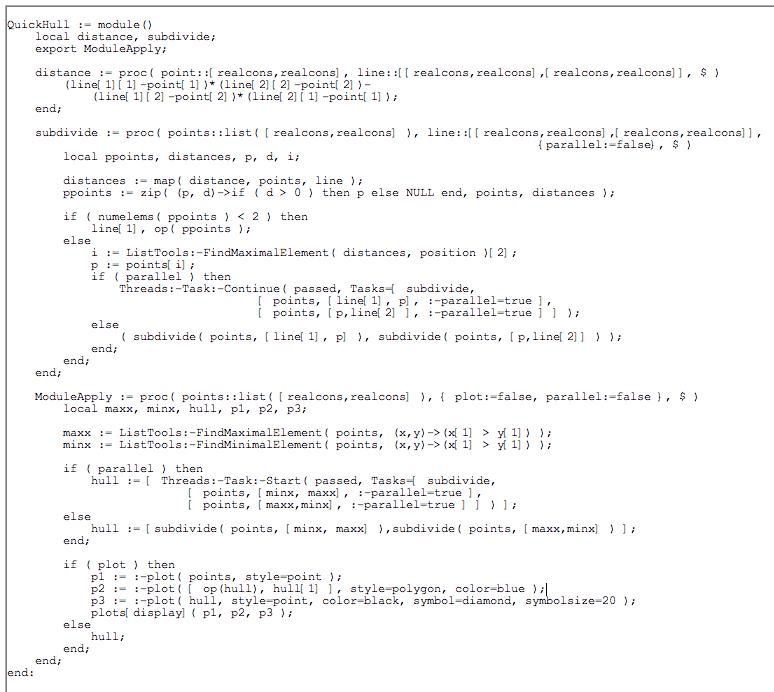Multithreaded Programming - Maple Features - Maplesoft

• Maple schedules the Tasks to the processors so that the code scales to the number of available processors.
• Multiple algorithms written using the Task Programming Model can run at the same time without significant performance impact.
• Complex problems can be solved without requiring traditional synchronization tools such as mutexes and condition variables.
• If such synchronization tools are not used, the function cannot be deadlocked.
• The Task functions are simple and the model mirrors conventional function calling.Here is an example of using this functionality to compute a convex hull. Given X, a set of points in 2-D, the convex hull is the minimum set of points that define a polygon containing all the points of X. If you imagine the points as pegs on a board, you can find the convex hull by surrounding the pegs by a loop of string and then tightening the string until there is no more slack.By adding parallelism, which was relatively simple to do, to the standard quick hull algorithm for computing convex hulls, you can see substantial speedups: